Courses

# Solved Subjective Questions - Stoichiometry, Class 11, Chemistry | EduRev Notes

## Class 11 : Solved Subjective Questions - Stoichiometry, Class 11, Chemistry | EduRev Notes

The document Solved Subjective Questions - Stoichiometry, Class 11, Chemistry | EduRev Notes is a part of the Class 11 Course Chemistry for JEE.
All you need of Class 11 at this link: Class 11

Ex.1 Calculate the following for 49 gm of H2SO4

(a) moles (b) Molecules (c) Total H atoms (d) Total O atoms (e) Total electrons

Sol. Molecular wt of H2SO4 = 98

(a) moles =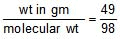=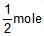(b) Since 1 mole = 6.023 × 1023 molecules.= 6.023 × 1023 × molecules = 3.011 × 1023 molecules

(c) 1 molecule of H2SO4 Contains 2 H atom

3.011 × 1023 of H2SO4 contain 2 × 3.011 × 1023 atoms = 6.023 × 1023 atoms

(d) 1 molecules of H2SO4 contains 4 O atoms

3.011 × 1023 molecular of H2SO4 contains = 4 × 3.011 × 1023 = 12.044 × 1023

(e) 1 molecule of H2SO4 contains 2H atoms 1 S atom 4 O atom

this means 1 molecule of H2SO4 Contains (2 16 4 × 8) e_

So 3.011 × 1023 molecules have 3.011 × 1023 × 50 electrons = 1.5055 × 1025 e_

Ex.2 Calculate the total ions & charge present in 4.2 gm of N_3

Sol. mole =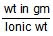=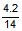= 0.3

total no of ions = 0.3 × NA ions

total charge = 0.3 NA × 3 × 1.6 × 10_19

= 0.3 × 6.023 × 1023 × 3 × 1.6 × 10_19 , = 8.67 × 104Ans.

Ex.3 Find the total number of iron atom present in 224 amu iron.

Sol. Since 56 amu = 1 atom

therefore 224 amu =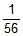× 224 = 4 atom Ans.

Ex.4 A compound containing Ca, C, N and S was subjected to quantitative analysis and formula mass determination. A 0.25 g of this compound was mixed with Na2CO3 to convert all Ca into 0.16 g CaCO3. A 0.115 gm sample of compound was carried through a series of reactions until all its S was changed into SO42_ and precipitated as 0.344 g of BaSO4. A 0.712 g sample was processed to liberated all of its N as NH3 and 0.155 g NH3 was obtained. The formula mass was found to be 156. Determine the empirical and molecular formula of the compound.

Sol. Moles of CaCO3 =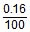= Moles of Ca

Wt of Ca =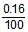× 40

Mass % of Ca =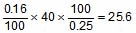Similarly Mass % of S =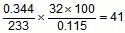Similarly Mass % of N = = 17.9

Þ Mass % of C = 15.48

Now :

Elements Ca S N C

Mass % 25.6 41 17.9 15.48

Mol ratio 0.64 1.28 1.28 1.29

Simple ratio 1 2 2 2

Empirical formula = CaC2N2S2,

Molecular formula wt = 156 , n × 156 = 156 Þ n = 1

Hence, molecular formula = CaC2N2S2

Ex.5 A polystyrne having formula Br3C6H3(C3 H8)n found to contain 10.46% of bromine by weight. Find the value of n. (At. wt. Br = 80)

Sol. Let the wt of compound is 100 gm & molecular wt is M

Then moles of compound =

Moles of Br = × 3

wt of Br = × 3 × 80 = 10.46

M = 2294.45 = 240 75 44 n , Hence n = 45 Ans.

Ex.6 A sample of clay was partially dried and then analysed to 50% silica and 7% water. The original clay contained 12% water. Find the percentage of silica in the original sample.

Sol. In the partially dried clay the total percentage of silica water = 57%. The rest of 43% must be some impurity. Therefore the ratio of wts. of silica to impurity = . This would be true in the original sample of silica.

The total percentage of silica impurity in the original sample is 88. If x is the percentage of silica, ; x = 47.3% Ans.

Ex.7 A mixture of CuSO4.5H2O and MgSO4. 7H2O was heated until all the water was driven-off. if 5.0 g of mixture gave 3 g of anhydrous salts, what was the percentage by mass of CuSO4.5H2O in the original mixture ?

Sol. Let the mixture contain x g CuSO4.5H2O

Þ = 3 Þ x = 3.56

Þ Mass percentage of CuSO4. 5H2O = = 71.25 % Ans.

Ex .8 367.5 gm KClO3 (M = 122.5) when heated, How many litre of oxygen gas is proudced at S.T.P.

Sol. KClO3 ® KCl O2

Applying POAC on O, moles of O in KClO3 = moles of O in O2

3 × moles of KClO3 = 2 × moles of O2

3 × = 2 × n, n = ×

Volume of O2 gas at S.T.P = moles × 22.4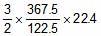= 9 × 11.2 = 100.8 lit Ans.

Ex.9 0.532 g of the chloroplatinate of a diacid base on ignition left 0.195 g of residue of Pt. Calculate molecular weight of the base (Pt = 195)

Sol. Suppose the diacid base is B.

B H2PtCl6® BH2PtCl6 ® Pt

diacid acid chloroplatinate

base 0.532 g 0.195 g

Since Pt atoms are conserved, applying POAC for Pt atoms,

moles of Pt atoms in BH2PtCl6 = moles of Pt atoms in the product

1 × moles of BH2PtCl6 = moles of Pt in the product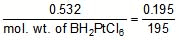mol. wt. of BH2PtCl6 = 532

From the formula BH2PtCl6, we get

mol. wt. of B = mol. wt. of BH2PtCl6 _ mol. wt. of H2PtCl6

= 532 _ 410 = 122. Ans.

Ex.10 10 mL of a gaseous organic compound containing. C, H and O only was mixed with 100 mL of oxygen and exploded under conditions which allowed the water formed to condense. The volume of the gas after explosion was 90 mL. On treatment with potash solution, a further contraction of 20 mL in volume was observed. Given that the vapour density of the compound is 23, deduce the molecular formula. All volume measurements were carried out under the same conditions.

Sol. CxHyOz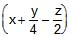O2 ® xCO2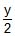H2O

10 ml

after explosion volume of gas = 90 ml

90 = volume of CO2 gas volume of unreacted O2

on treatment with KOH solution volume reduces by 20 ml. This means the volume of CO2 = 20 ml

the volume of unreacted O2 = 70 ml

volume of reacted O2 = 30 ml

V.D of compoud = 23

molecular wt 12x y 16z = 46 ...(1)

from equation we can write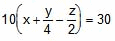, x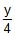_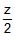= 3

4x y _ 2z = 12 ...(2)

& 10x = 20 Þ x = 2

from eq. (1) & (2) ; z = 1 & y = 6; Hence C2H6Ans.

Offer running on EduRev: Apply code STAYHOME200 to get INR 200 off on our premium plan EduRev Infinity!

## Chemistry for JEE

223 videos|452 docs|334 tests

,

,

,

,

,

,

,

,

,

,

,

,

,

,

,

,

,

,

,

,

,

,

,

,

,

,

,

;